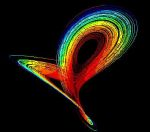# Science Quiz / Fork Lightning Math 2

Random Science or Math Quiz

## Can you name the numbers that make up the math chains?

#### bywonderslime  Plays Quiz not verified by Sporcle

How to Play
Score
0/40
Timer
10:00
Next Operation:Value:Bonus Clues:
#1: Start by entering '10'.
#3: Multiply that by 2.
#4: Add the digits of that answer together and then multiply by 2.
#5: Take three-quarters of that.
#6: Subtract 17.9 from that.
#7: Multiply that by 300.
#9: Subtract 3456.
#10: Divide that by three to get...!
Next Operation:Value:Bonus Clues:
#11: Use the bonus clue from Question #5.
#12: Add to that the number of nickels in a dime.
#13: Divide by the number of dimes in \$1.60.
#14: Add to that the amount of change you would get for paying \$10 for a \$7.75 item.
#15: Subtract the value in dollars of nine quarters.
#16: Multiply that by the number of Canadian loonies whose value equals that of one toonie.
#17: Multiply by the number of nickels in \$2.10.
#18: Add the number of farthings that used to make up an English penny.
#19: Add the number of pennies in a single standard roll.
#20: If your answer to #19 is the price of an item and 12.5% tax is applied, what is the total cost?
Next Operation:Value:Bonus Clues:
#21: Use the bonus clue from Question #7.
#22: Add to that the number of seconds in a half-minute.
#23: Add the number of years that made up the 2nd Century.
#24: Divide by the number of minutes it takes to get from 1:42 pm to 2:10 pm.
#25: Divide by the number of decades in ten millennia.
#26: Multiply by the time in minutes it takes for a full one-hour hourglass to empty.
#27: Add the time in seconds it takes for that hourglass to empty again from full when flipped.
#28: Subtract from that the number of hours in a week.
#29: Subtract from that the total number of seconds you were given to do this quiz.
#30: If the answer to #29 is a number of days, then how many complete fortnights are there in that period of time?
Next Operation:Value:Bonus Clues:
#31: Use the bonus clue from Question #25.
#33: Divide that by III.
#35: Multiply by the square root of XVI.
#37: Divide by IV.
#38: Add the largest number out of all previous correct responses in this quiz.
#39: What is the remainder after dividing that number by XVI?
#40: If you write the four hidden keys of this quiz out in order from left to right, you get an eight-digit number in binary. What is its corresponding decimal expansion?

## From the Vault See Another

Pearl Jam Songs

by Ben

Music 15m
In preparation for their ninth studio album, take a moment to relive their first eight.

### Extras

Created Dec 10, 2010
Tags: